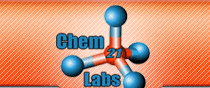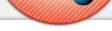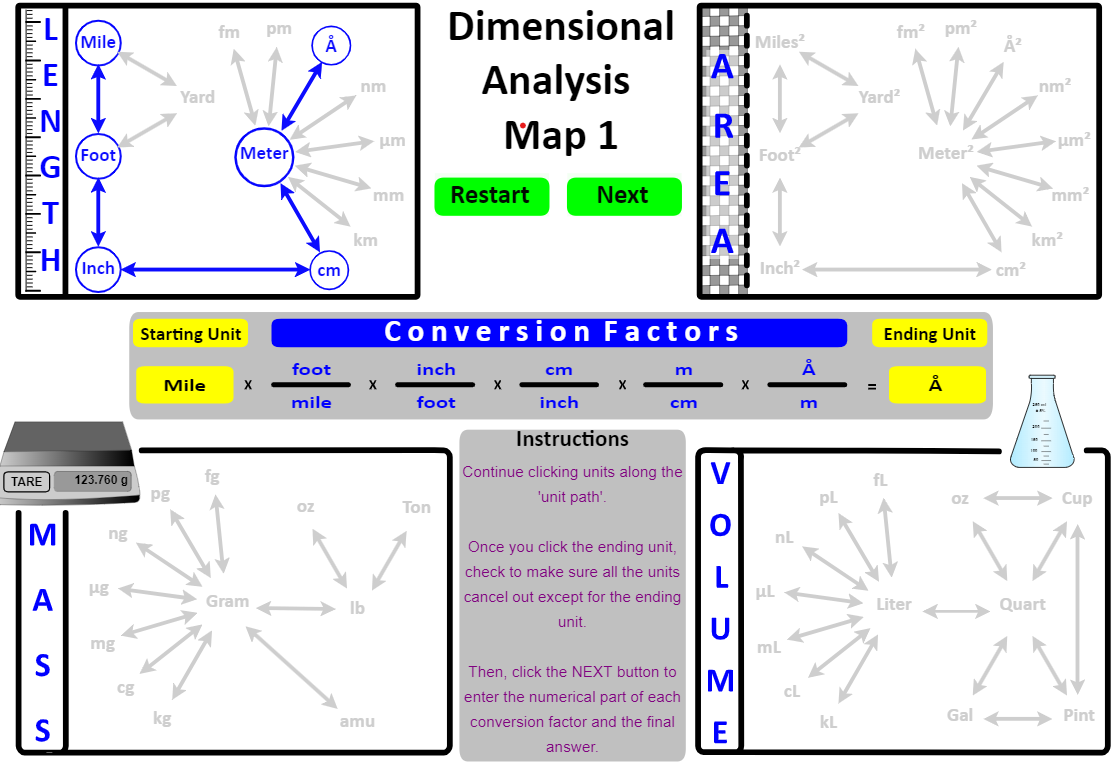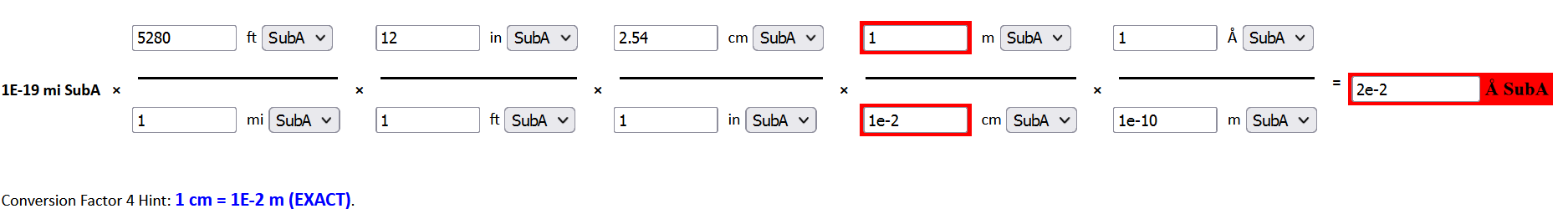Online Lab Submission Students: Instructors: Digital Labs Testimonials Royalties Embedding Content Images, Videos, Webpages, Animations Interactive Spectra Grading Essays / Images Web Reports Display Student Lab Data and Results Automatic Grading of Reported Precision of Lab Data (Decimal Places) Automatic Grading of Reported Significant Figures of Lab Calculations Automatic Grading of Accuracy and Precision of Lab Results Lab Practical / Snow Day / Lab Final Cheating Virtual Labs: Pre-Lab Assignments Interactive Animations: Teaching Dimensional Analysis - Interactive Map of Units To be successful in chemistry, write your work down - don't do it in your head, even with the aid of a calculator. Make sure any numbers have associated units and labels (i.e., 1.23 g H2O). Numbers have no meaning without their units of measurement . . . . 2 can be greater than 12 (2 kg is greater than 12 g). In general chemistry, students encounter measurements of all sorts . . . . time, temperature, mass, volume, length, moles, etc. . . . . and dimensional analysis allows the seamless conversion between units. Chem21Labs has developed a series of interactive unit maps that extend and build on the previous map and are used as active learning experiences to enhance one's understanding of dimensional analysis. Maps 1 and 2 are shown for demonstration purposes. Map 1: Length, Area, Mass and Volume Map 2: Volume, Mass, Density, Moles, Molar Mass, and Atoms Map 3: Map 2 is "duplicated" and placed to the right of the original Map 2 - the left side is Substance A and the right side is Substance B. The coefficient ratios from the balanced equation provide a "bridge" between Substance A units and Substance B units Map 4: Molarity and PV=nRT is added to Map 3 Many users have converted their first and second CHEM1 labs into labs where students work Dimensional Analysis problems. The first lab consists of 10 problems that require students to use Map 1 to arrive at the answers . . . the second lab contains another 10 problems and uses Map 2. The use of interactive dimensional analysis maps in lab has several advantages: Students are not ready for a wet lab. Students that miss the first lab can easily make it up because it can be performed out-of-class. Also, the 2nd lab can be used to check-in to lab desks and use the remaining time to work the Map 2 dimensional analysis problems. Students that have learned the ratio method in high school are forced to learn the factor-label method which will be invaluable to them in university science courses. The lab setting is a more intimate environment to help students who struggle with dimensional analysis problems. As the more proficient students finish and leave the laboratory, one-on-one help can be directed toward the remaining students. This is a perfect time in the semester to ensure that students can . . . . use their calculators - especially in entering numbers in scientific notation with the EE or EXP buttons determine the number of significant figures in the problem and enter their answer expressed to the correct number of significant figures Map 1: Length, Area, Mass and Volume Map Click on the starting unit.Find a path between the starting unit and ending unit and click the units starting with the one adjacent to the starting unit. This sets up the factor-label part of the dimensional analysis problem. If the problem wanted you to convert 1E-19 miles into Å, you would click on the following units in the order listed:  mile, foot, inch, cm, m, Å .Go ahead and click these units in the interactive map below. If the units were clicked as described, your map will look like . . . .Notice that the units have been "set up" in the middle of the map: it starts with  mile  and ends with  Å  and all the units cancel out except  Å . In the "live" implementation, the student would click the Next button to open a web page that has the "numerical part" empty awaiting entry by the student. In the image below, the student has entered everything correctly except the conversion between cm and m.Items entered incorrectly are displayed back to the student in  red . In addition, the correct conversion factor is listed at the bottom of the image in blue text. Instead of hoping that students create their own "map" of units and their relationships, this interactive assignment "guides" the student through the process.  As with any map, the more it is used, the less it needs to be used.  Map 2: Volume, Mass, Density, Moles, Molar Mass, and Atoms Determine if the problem is asking you to report a numeric answer for a "1-unit" problem or a "2-unit" problem (density (g/mL or g/L) or molar mass (g/mole). One Unit Click on the starting unit. Find a path between the starting unit and ending unit and click the units starting with the one adjacent to the starting unit. This sets up the factor-label part of the dimensional analysis problem. In the "live" implementation, the student would click the Next button to open a web page where she would enter the "numerical part" of the conversion factors and the final answer. Two Unit: the answer to the problem is density or molar mass. Click the Two Unit button - this opens a factor-label method in the numerator AND denominator at the bottom of the Map Suppose the question said "10.5 pg of a substance has a volume of 65.7Å3. What is it's density in ng/L?". Click on pg - the starting numerator unit. Find a path between pg and ng (the ending numerator unit). Click the Denominator button and click on Å3. Find a path between Å3 and L (the ending denominator unit). In the "live" implementation, the student would click the Next button to open a web page where she would enter the "numerical part" of the conversion factors and the final answer.